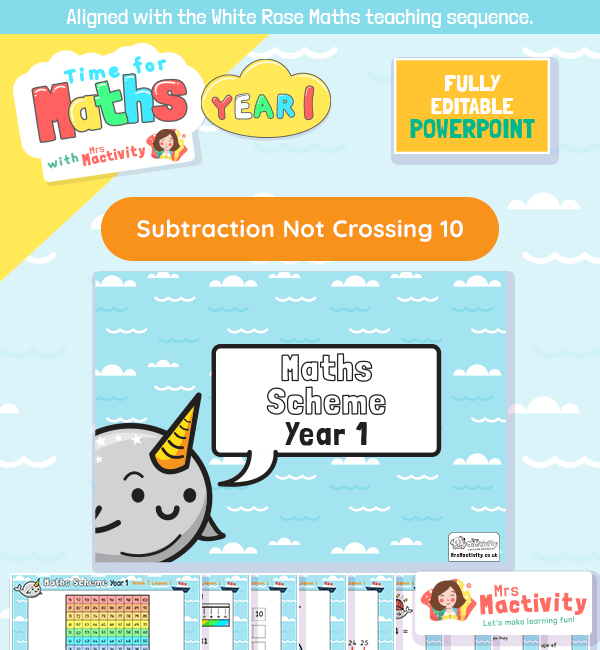# Subtraction Not Crossing 10 Lesson Presentation## White Rose Maths subtraction not crossing 10 resources

Aligned with the White Rose Maths scheme of work, this Subtraction Not Crossing 10 Lesson Presentation is fully editable, and is designed for the year 1 maths curriculum covering the following maths objectives for the spring term:

• Identify and represent numbers using objects and pictorial representations including the number line, and use the language of: equal to, more than, less than (fewer), most, least
• Read and write numbers from 1 to 20 in numerals and words
• Solve one-step problems that involve addition and subtraction, using concrete objects and pictorial representations, and missing number problems such as 7=_ 9

Aligned with the order of teaching of the White Rose Maths scheme of work, use this to help your children get to grips with subtraction. Includes maths continuous provision ideas and non-worksheet maths learning too.

Explore our maths mastery resources.

Explore our year 1 maths resources.

Explore our addition and subtraction resources.